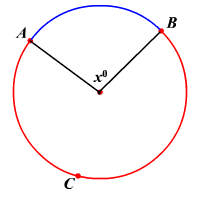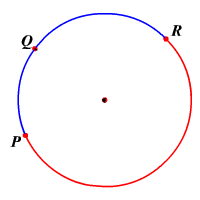# Arcs

A minor arc is the shorter arc connecting two endpoints on a circle .

The measure of a minor arc is less than $180°$ , and equal to the measure of the arc's central angle .

A major arc is the longer arc connecting two endpoints on a circle.

The measure of a major arc is greater than $180°$ , and equal to $360°$ minus the measure of the minor arc with the same endpoints.

An arc measuring exactly $180°$ is called a semicircle .In the figure, $m\stackrel{⌢}{AB}=x°$ and $m\stackrel{⌢}{ACB}=\left(360-x\right)°$ .

Note that the minor arc is named with just two endpoints, while the major arc is named with three letters, the two endpoints and a third point on the arc.

Adjacent arcs are arcs of the same circle that have exactly one point in common.In the circle, $\stackrel{⌢}{PQ}$ and $\stackrel{⌢}{QR}$ are adjacent arcs.
So, $m\stackrel{⌢}{PQR}=m\stackrel{⌢}{PQ}+m\stackrel{⌢}{QR}$ .